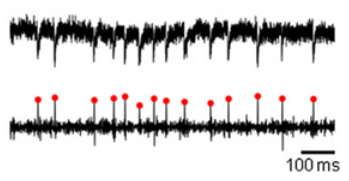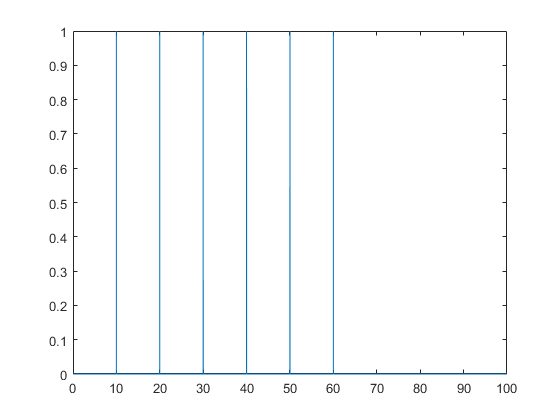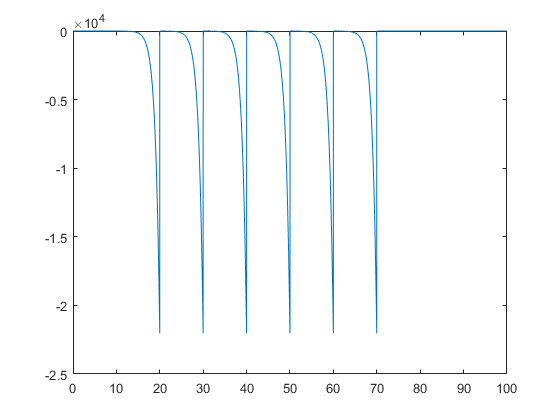# Deconvolutions and EPSCs

So at the research lab I’m working in this summer, the field of study is in neuroscience. And while that’s relatively new and unknown territory for me, I have tried my best to learn and to bring my own experiences and skills to the new problems I face.

## EPSCs

Part of what I’m doing involves investigating electrical properties of neurons in the spinal cord. Neurons communicate with each other at junctions called chemical synapses. At these junctions, neurotransmitters are released from one neuron (the presynaptic neuron), which causes a change in the receptors on the postsynaptic neuron, causing a flow of ions into the cell. In this way, a neuron receives inputs from many other neurons.

To look at these inputs, the voltage clamp technique is used to hold the membrane potential at a constant level. The current required to keep the constant potential is measured, and the different inputs are represented by spikes, the excitatory postsysnaptic currents (EPSCs). By analyzing the characteristics of these EPSCs (such as the shape of these spikes and their frequencies), we can start to understand the functions of different neurons.Simulated voltage clamp data

## Deconvolutions

However, manual detection and analysis of these EPSCs is tedious and subject to observer bias. In this paper by Pernía-Andrade et al, a method of detecting EPSCs is proposed using deconvolutions.

Now, if you don’t know what a deconvolution is (or a convolution, for that matter), don’t worry (I’m looking at you, future Edward). I’ll try and break it down here, but I can’t promise I’ll do a good job. First, we’ll start with a description of linear time-invariant systems (LTI). An LTI is a system that is linear: meaning that the output of the system is a linear map (where addition and scalar multiplication are conserved). So if I have an input $x_1(t)$ with an output $y_1(t)$, and an input $x_2(t) \to y_2(t)$, then $3x_1(t) \to 3y_1(t)$, and $x_1(t) + x_2(t) \to y_1(t) + y_2(t)$.

The second property is time-invariance. This is much easier to describe intuitively: it simply means that the output of a given input does not vary with time. Formally, if $x(t) \to y(t)$, then $x(t - t_0) \to y(t - t_0)$.

So let’s model our neuron (as described above) as an LTI. Our input signal would be a release of neurotransmitters into the synapse. Our output, or impulse response, is the change electrical potential in the cell. Mathematically, we can model these inputs as delta functions. Since in voltage clamp, we are holding the membrane potential, then a negative current would be detected to counteract the influx of ions. In short, this is the EPSC. Let’s assume we can model this with an exponential function.

Now, given that cells receive many signals over time, how can we get the full electrical activity of the cell? You can imagine that this repeated signalling would be a series of delta functions.Delta function impulses

We know that each delta function has an impulse response modelled with a exponential function. In the case where these signals are spaced out enough, the overall output signal would just be a series of exponentials corresponding to each impulse (see what I did there?).Output function with exponential impulse response

Easy. But what if these impulses were closer to each other? Then the decay of the exponential response would overlap with the rise of the next response, and the resulting signal would not be as easy to find.

This is where convolutions come in. We can get the resulting signal simply by convolving the input signal with the impulse response function. Conversely, by deconvolving an output signal (such as our voltage clamp trace) with the impulse response function, we can get the input signal!

## Tying it all together

Pernía-Andrade et al basically did what we just walked through: he modelled the electrical activity of the neuron as an LTI, and found the input signal (the series of EPSCs) by deconvolving the output (the voltage clamp trace) by the impulse response function (he used a biexponential function). This works out pretty well because in reality, the system is pretty much an LTI:

• It’s linear, meaning that the EPSC grows linearly with the amount of neurotransmitters released (at least up to a certain threshold that wouldn’t normally be reached in the cell),

• It’s time-invariant, as the EPSC generated due to an input is the same regardless of when the input is received. It can be argued that experimentally, the cell could start to die during the recording, compromising the activity of the neurotransmitters at the synapse, but for most cases I think the LTI model is accurate.

The paper goes on to derive a detection threshold for the deconvolved signal by modelling this signal as a Gaussian distribution and making their threshold ($\theta$) 4-4.5 times the $\sigma$ of the distribution. I’m not quite sure how they got this to be honest.

## Some problems

While I think that this paper is really cool and has some real application to the analysis I’m doing, there are some problems.

• Noise isn’t really considered, and in these types of recordings the noise tends to take shapes that are very similar to EPSCs. While this doesn’t really matter if you’re only looking for high amplitude EPSCs, my research requires the detection of EPSCs that are of comparable magnitude to the noise detected.

• This technique works really well if your template function is quite accurate: in my own work, I was blown away by how effective it was. However, the template function I used was an average of manually detected EPSCs. While this can still be used to calculate frequencies in a more quantitative way, it still depends on an accurate template that is subject to human bias.

This paper by Ando-Ardó et al describes a way to get these template functions using more sophisticated statistics. It’s interesting that this paper is actually published before the one discussed before; nevertheless it’s definitely not as accessible because of how involved the math is. I haven’t even wrapped my head around it. Merel et al skipped the deconvolution step completely, using Bayesian probability to detect the EPSCs after mathematically modelling the voltage clamp. Both of these papers present further directions that may help my own analysis…alas I don’t get all the math yet.